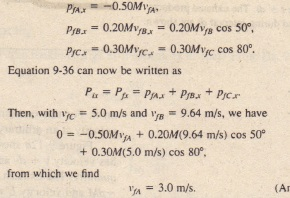# Sample Problem

Sample Problem

A firecracker placed inside a coconut of mass M. initially at rest on a friction less floor. blows the coconut into three pieces that slide across the floor. An overhead view is shown in Fig. 9-1Oa. Piece C. with mass O.30M. has final speed tc = 5.0 m

(a) What is the speed of piece 8. with mass 0.20M?

SOLUTION: A Key Idea here is to see whether linear momentum is conserved. We note that (I) the coconut and its pieces form a closed system, (2) the explosion forces are internal to that system. and (3) no net external force acts on the system. Therefore. the linear momentum of the system is conserved.

To get started, we superimpose an coordinate system as shown with the negative direction of the x axis coinciding with the direction . The x axis is at 80° with the direction of c and 50° with the direction.

A second Key Idea is that linear momentum is conserved separately along both x and y axes. Let’s use the y axis and writeThree pieces of an explodedcoconut move off in three directions along a friction less floor.(a) An overhead view of the event. (b) The same with a two dimensionalaxis system imposed.

1» What is the speed of piece A?

SOLUTION . Because linear momentum is also conserved along the axis,

we find the x components of the final momenta, using the fact that , piece A must have a mass .CHECKPOINT 6: Suppose that the exploding coconut is ac- SOLUTION . Because linear momentum is also conserved along the x celebrating in the negative direction (say It IS on axis, we have  a ramp that slants downward In that direction). Is linear men tum conserved along (a) the .r axis (as stated in ) and’here Pi, = 0 because the coconut is initially at rest. To get PI” (b) the y axis (as stated)?